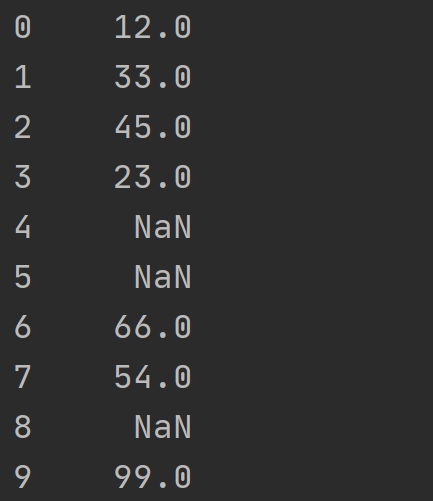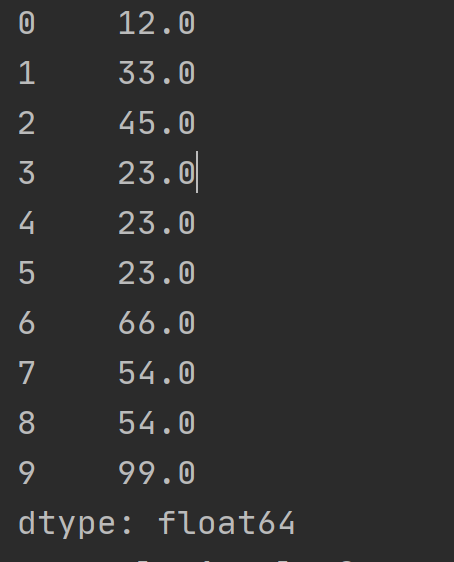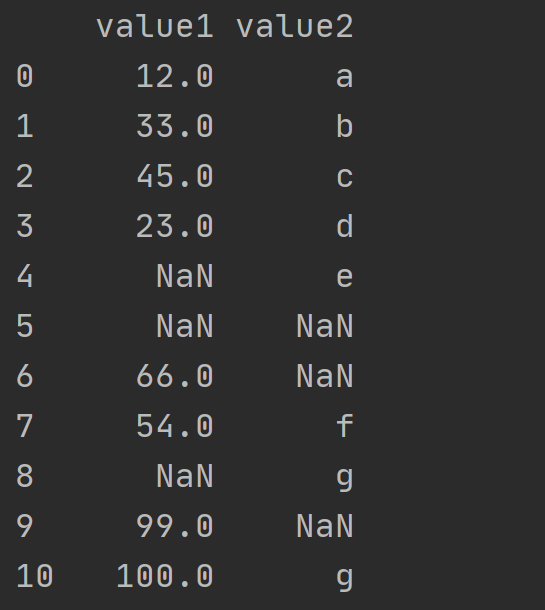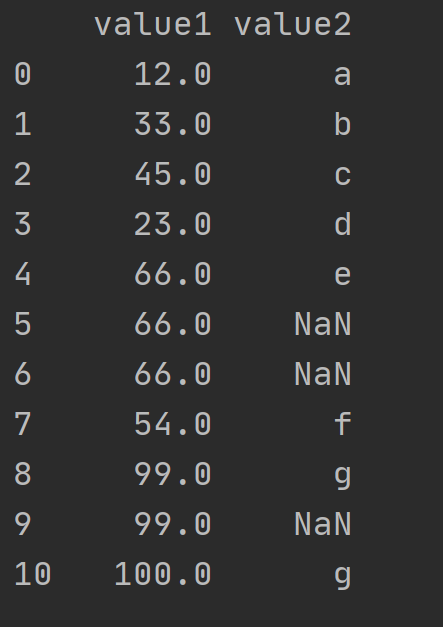• dropna()删除缺失值
2022-05-12 10:42:01

删除包含缺失值的行

.dropna()

删除所有值都缺失的行

.dropna(how=‘all’)

删除至少2个值缺失的行

.dropna(thresh=2)

删除包含缺失值的列

.dropna(1)apython
更多相关内容
• 通过dropna函数删除带有缺失数据的行或列
import numpy as np
import pandas as pd

#  创建一个数据
# np.nan 表示空值 NaN
# 鸢尾花的数据结构：
'''
	Sepal.Length（花萼长度）；
	Sepal.Width（花萼宽度）；
	Petal.Length（花瓣长度）；
	Petal.Width（花瓣宽度））。
'''

data = pd.DataFrame({"Sepal_Length":[3, 5.6, 6],
"Sepal_Width":[2, 3.5, 4.5],
"petal_length":[1, 1.4, 6.5],
"Petal_Width":[np.nan, np.nan, 0.5]})
print(data)

# 当该行数据全为空时，删除该行数据
# df = data.dropna(axis=0, how="all", thresh=None) # axis = 0行 1列；how = "all" 如果所有值为空，该行数据删除  “any” 任意值为空
# print(df)
#
# # 如果该行存在缺失值，则删除该行数据
# df = data.dropna(axis=0, how="any", thresh=None)
# print(df)

# 80% 法则 当缺失数据超过20%情况，删除该行或该列
num = 3
df = data.dropna(axis=1, thresh=num * 0.8)
print(df)

展开全文python 机器学习 数据挖掘
• 2.2 构建一个DataFrame 删除空值数量≥3的行df.dropna(thresh=3)删除索引为2的行中，存在空值的列df.dropna(subset=,axis=1) 结果为：

# 一、函数参数

• 函数形式：dropna(axis=0, how=‘any’, thresh=None, subset=None, inplace=False)
• 参数：
axis：0或’index’，表示按行删除；1或’columns’，表示按列删除。
how：‘any’，表示该行/列只要有一个以上的空值，就删除该行/列；‘all’，表示该行/列全部都为空值，就删除该行/列。
thresh：int型，默认为None。如果该行/列中，非空元素数量小于这个值，就删除该行/列。
subset：子集。列表&
展开全文python 开发语言
• 以上这篇python 检查数据中是否有缺失值,删除缺失值的方式就是小编分享给大家的全部内容了，希望能给大家一个参考，也希望大家多多支持软件开发网。 您可能感兴趣的文章:python实现数据清洗(缺失值与异常值处理)...python 数据
• np.nan,np.nan,'f','g',np.nan,'g']}) print(s) s.ffill(0,inplace=True) print(s) print(df) ''' method：pad/ffill:用前面的填充 backfill/bfill:用后面的填充 ''' df["value1"].fillna(method='backfill',in...
import  numpy as np
import pandas as pd
s=pd.Series([12,33,45,23,np.nan,np.nan,66,54,np.nan,99])
df=pd.DataFrame({'value1':[12,33,45,23,np.nan,np.nan,66,54,np.nan,99,100],
'value2':['a','b','c','d','e',np.nan,np.nan,'f','g',np.nan,'g']})
print(s)
s.ffill(0,inplace=True)
print(s)
print(df)
'''
backfill/bfill:用后面的值填充
'''
df["value1"].fillna(method='backfill',inplace=True)
print(df)展开全文python
• pandas使用dropna函数删除dataframe中列缺失值的个数大于某一比例阈值的数据列（drop columns with missing values proportion more than a threshold in dataframe）数据挖掘 python 人工智能
• 【小白从小学Python、C、Java】【Python全国计算机等级考试】【Python数据分析考试必会题】● 标题与摘要Python中缺失值删除pd.dropna()函数● 选择题以下关于dropna()函数说法错误的是：A 用于删除缺失数据B axis=0...python 数据挖掘 mysql 程序人生
• pandas使用dropna函数删除dataframe中列缺失值的个数大于某一阈值的数据列（drop columns with missing values more than a threshold in dataframe） 目录python 数据挖掘 人工智能
• 应用dropna实现缺失值删除 应用replace实现数据的替换 1 如何处理nan 获取缺失值的标记方式(NaN或者其他标记方式) 如果缺失值的标记方式是NaN 判断数据中是否包含NaN： pd.isnull(df), pd.notnull(df) ...isnull fillna replace
• 函数形式：dropna(axis=0, how=‘any’, thresh=None, subset=None, inplace=False) 参数：    axis：0或’index’，表示按行删除；1或’columns’，表示按列删除。    how：‘any’，表示该行/列只要有一个以上...python
• [Pandas] 缺失值删除 df.dropna()方法python
• 使用dropna（）方法删除缺失值，使用fillna（）方法用其他值替换（填充）缺失值。 如果要提取包含缺失值的行或列，使用isnull（）方法确定元素是否缺失。 例如，读取并使用包含带read_csv的空格的csv文件。 import ...python 机器学习
• 缺失值的个数只占整体很小一部分的时候，可直接删除缺失值（行）。但是如果缺失值占比比较大，这种直接删除缺失值的处理方法就会丢失重要信息。 直接删除法处理缺失值时，需要检测样本总体中缺失值的个数。Python...
• pandas使用dropna函数删除dataframe中全是缺失值的数据列（dropcolumns with all missing values in dataframe）数据挖掘 python 人工智能
• D, dtype: float64 要绘制没有数据为NaN的日期,你可以这样做： fig, ax = plt.subplots() ax.plot(range(vals.dropna().size), vals.dropna()) ax.set_xticklabels(vals.dropna().index.date.tolist()); fig....
• 主要给大家介绍了关于Python Pandas对缺失值的处理方法，文中通过示例代码介绍的非常详细，对大家学习或者使用Python Pandas具有一定的参考学习价值，需要的朋友们下面来一起学习学习吧
• pandas使用dropna函数删除dataframe中所有包含缺失值的数据行（drop rows which contain missing vlaues in dataframe）python 数据挖掘 人工智能
• pandas使用dropna函数删除dataframe中列非缺失值的个数小于某一比例阈值的数据列（drop columns with non missing values less than a proportion threshold in dataframe）数据挖掘 python 人工智能
• ## pandas || df.dropna() 缺失值删除

万次阅读 多人点赞 2020-09-01 10:55:25
df.dropna()函数用于删除dataframe数据中的缺失数据，即 删除NaN数据. 官方函数说明： DataFrame.dropna(axis=0, how='any', thresh=None, subset=None, inplace=False) Remove missing values. See the User ...python 数据挖掘 机器学习
• df.dropna()#直接删除含有缺失值的行 df.dropna(axis = 1)#直接删除含有缺失值的列 df.dropna(how = ‘all’)#只删除全是缺失值的行 df.dropna(thresh = 4)#保留至少有4个缺失值的行 df.dropna(subset = [‘C’])#...
• 面对缺失值三种处理方法： option 1： 去掉含有缺失值的样本（行） option 2：将含有缺失值的列（特征向量）去掉 option 3：将缺失值用某些值填充（0，平均值，中值等） 对于dropna和fillna,dataframe和series都有...Python
• 在实际应用中对于数据进行分析的时候，经常能看见缺失值，下面来介绍一下如何利用pandas来处理缺失值。常见的缺失值处理方式有，过滤、填充。 缺失值的判断 pandas使用浮点值NaN(Not a Number)表示浮点数和非浮点...
• 删除缺失值 dropna()：删除缺失的值。 返回值：DataFrame 删除了NA条目的DataFrame。不改变原序列值。 参数检验： axis ： {0或’index’，1或’columns’}，默认0 确定是否删除包含缺失值的行或列。 0或’index...
• ## Pandas剔除缺失值

千次阅读 热门讨论 2021-11-16 10:26:12
缺失值处理方法，包括 dropna()（剔除缺失值）和 fillna()（填充缺失值）。本文介绍如何利用 Pandas 剔除缺失值python 机器学习 数据挖掘
• Python Pandas缺失值处理 在一些数据分析业务中，数据缺失是我们经常遇见的问题，缺失值会导致数据质量的下降，从而影响模型预测的准确性，这对于机器学习和数据挖掘影响尤为严重。因此妥善的处理缺失值能够使模型...
• 参考资料 ...函数功能：当DataFrame数据中出现缺失值时，删除这些缺失值所在的轴（满足特定条件） 调用方法： df= data.dropna(axis=0,how='any',thresh=None,subset=None,inplace=False) 参数...python panda
• 缺失值可能是数据科学中最不受欢迎的值，然而，它们总是在身边。忽略缺失值也是不合理的，因此我们需要找到有效且适当地处理它们的方法。 在本文中，我们将介绍 8 种不同的方法来解决缺失值问题，哪种方法最适合特定...python 数据挖掘...